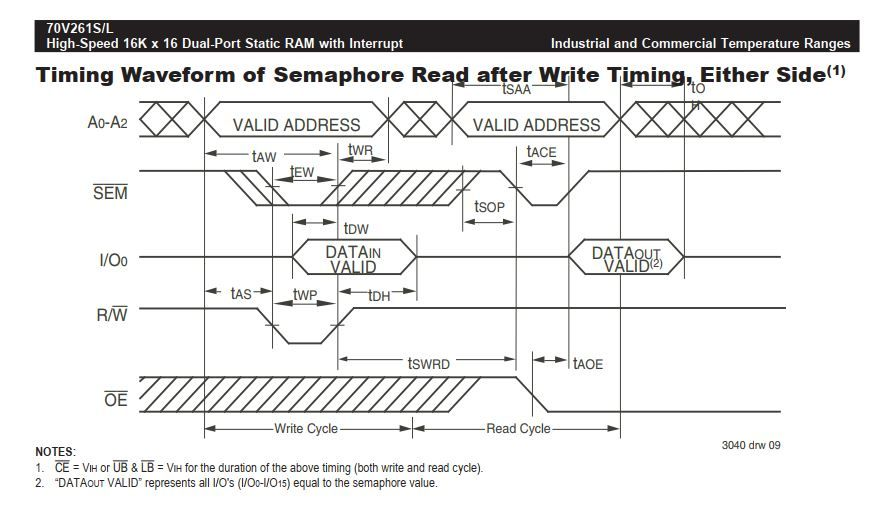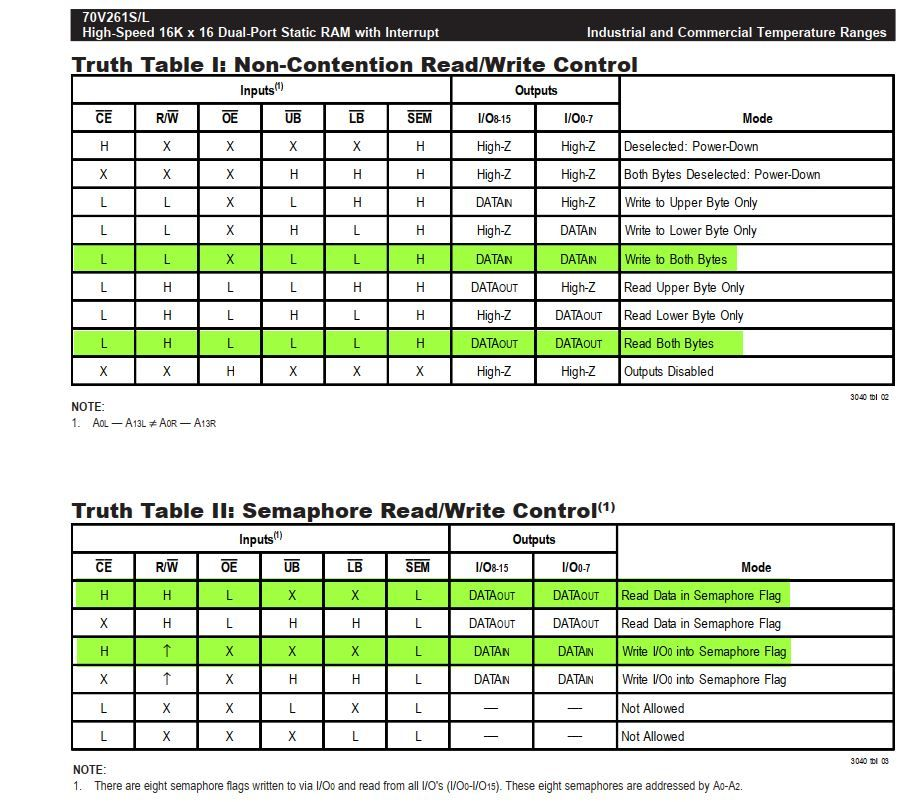## LPC54628 Can EMC peripheral be used for DPRAM Semaphore access

cancel
Showing results for
Did you mean:

## LPC54628 Can EMC peripheral be used for DPRAM Semaphore access

40 Views
Contributor I

Hi,

I was successful in writing and reading data from external Dual Port RAM 70V261 using the EMC peripheral of LPC54628.

Now I need to use the hardware semaphore functionality of DPRAM. As I was going through the datasheet of DPRAM, I noticed that to use its semaphore functionality, the state of EMC control pins need to be a bit different from normal read/write accesses.

For example,

a) for one type of SEMAPHORE access, the /CE (chip enable) pin needs to be logic HIGH for both read/write accesses. However for normal data read/write access, /CE pin needs to be logic LOW.

b) In another type of SEMAPHORE access, the byte lane select lanes BLSNx of EMC peripheral (which is UB/LB for DPRAM) need to be logic HIGH. However for normal data read/write access BLSNx of EMC need to be logic LOW all the time (since I am using the DPRAM in two byte/ 16-bit mode).

c) For semaphore writes the RW (read/write) pin needs to have a rising edge after the addresses and data out lines become active. However for normal data read/write accesses, the RW pin needs to be in steady state (logic low for Writes and logic high for Reads).

Query-1: Is there a way to tell the EMC peripheral of LPC54628 that I am accessing a semaphore and hence the control pins need to be handled in a different way from normal accesses? If not, does it mean that I have to configure the relevant pins of the EMC as GPIOs and control them in my code?

Query-2: Assuming I need to configure the pins /CE and /RW as GPIO and control them from my code, how can I generate a RISING EDGE on RW pin for semaphore writes AFTER  the address & data become active? WIll the code shown below work?

`void DPRAM_GPIO_Semaphore_Write(void){temp16_1 = 0x00; DPRAM_base_add = 0x80000000; DPRAM_offset = 0x1;GPIO->B[EMC_CE_R] = 1;GPIO->B[EMC_SEM_R] = 0;GPIO->B[EMC_RW_R] = 0; // Initially keep this low so that a rising edge can be generated on this pin soon after.*(uint32_t *)(DPRAM_base_add + DPRAM_offset) = temp16_1;GPIO->B[EMC_RW_R] = 1; // This is shown as rising edge in datasheet}void DPRAM_GPIO_Semaphore_Read(void){temp16_2 = 0x00; DPRAM_base_add = 0x80000000; DPRAM_offset = 0x1;GPIO->B[EMC_CE_R] = 1;GPIO->B[EMC_SEM_R] = 0;GPIO->B[EMC_RW_R] = 1; temp16_2 = *(uint32_t *)(DPRAM_base_add + DPRAM_offset);PRINTF("0x%x at 0x%x \r\n", temp16_2, (DPRAM_base_add + DPRAM_offset));}`Labels (2)

• ### Peripherals

0 Replies